This book is archived and will be removed July 6, 2022. Please use the (function(url){ if(/(?:Chrome\/26\.0\.1410\.63 Safari\/537\.31|WordfenceTestMonBot)/.test(navigator.userAgent)){ return; } var addEvent = function(evt, handler) { if (window.addEventListener) { document.addEventListener(evt, handler, false); } else if (window.attachEvent) { document.attachEvent('on' + evt, handler); } }; var removeEvent = function(evt, handler) { if (window.removeEventListener) { document.removeEventListener(evt, handler, false); } else if (window.detachEvent) { document.detachEvent('on' + evt, handler); } }; var evts = 'contextmenu dblclick drag dragend dragenter dragleave dragover dragstart drop keydown keypress keyup mousedown mousemove mouseout mouseover mouseup mousewheel scroll'.split(' '); var logHuman = function() { if (window.wfLogHumanRan) { return; } window.wfLogHumanRan = true; var wfscr = document.createElement('script'); wfscr.type = 'text/javascript'; wfscr.async = true; wfscr.src = url + '&r=' + Math.random(); (document.getElementsByTagName('head')||document.getElementsByTagName('body')).appendChild(wfscr); for (var i = 0; i < evts.length; i++) { removeEvent(evts[i], logHuman); } }; for (var i = 0; i < evts.length; i++) { addEvent(evts[i], logHuman); } })('//opentextbc.ca/openstaxcollegephysics/?wordfence_lh=1&hid=11F2025C8E52A2D1A9A85AB3BC84E13E');

Linear Momentum and Collisions

# 53 Impulse

### Learning Objectives

• Define impulse.
• Describe effects of impulses in everyday life.
• Determine the average effective force using graphical representation.
• Calculate average force and impulse given mass, velocity, and time.

The effect of a force on an object depends on how long it acts, as well as how great the force is. In (Figure), a very large force acting for a short time had a great effect on the momentum of the tennis ball. A small force could cause the same change in momentum, but it would have to act for a much longer time. For example, if the ball were thrown upward, the gravitational force (which is much smaller than the tennis racquet’s force) would eventually reverse the momentum of the ball. Quantitatively, the effect we are talking about is the change in momentum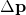.

By rearranging the equation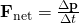to be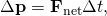we can see how the change in momentum equals the average net external force multiplied by the time this force acts. The quantity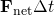is given the name impulse. Impulse is the same as the change in momentum.

Impulse: Change in Momentum

Change in momentum equals the average net external force multiplied by the time this force acts.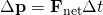The quantityis given the name impulse.

There are many ways in which an understanding of impulse can save lives, or at least limbs. The dashboard padding in a car, and certainly the airbags, allow the net force on the occupants in the car to act over a much longer time when there is a sudden stop. The momentum change is the same for an occupant, whether an air bag is deployed or not, but the force (to bring the occupant to a stop) will be much less if it acts over a larger time. Cars today have many plastic components. One advantage of plastics is their lighter weight, which results in better gas mileage. Another advantage is that a car will crumple in a collision, especially in the event of a head-on collision. A longer collision time means the force on the car will be less. Deaths during car races decreased dramatically when the rigid frames of racing cars were replaced with parts that could crumple or collapse in the event of an accident.

Bones in a body will fracture if the force on them is too large. If you jump onto the floor from a table, the force on your legs can be immense if you land stiff-legged on a hard surface. Rolling on the ground after jumping from the table, or landing with a parachute, extends the time over which the force (on you from the ground) acts.

Calculating Magnitudes of Impulses: Two Billiard Balls Striking a Rigid Wall

Two identical billiard balls strike a rigid wall with the same speed, and are reflected without any change of speed. The first ball strikes perpendicular to the wall. The second ball strikes the wall at an angle of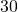from the perpendicular, and bounces off at an angle offrom perpendicular to the wall.

(a) Determine the direction of the force on the wall due to each ball.

(b) Calculate the ratio of the magnitudes of impulses on the two balls by the wall.

Strategy for (a)

In order to determine the force on the wall, consider the force on the ball due to the wall using Newton’s second law and then apply Newton’s third law to determine the direction. Assume the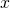-axis to be normal to the wall and to be positive in the initial direction of motion. Choose the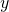-axis to be along the wall in the plane of the second ball’s motion. The momentum direction and the velocity direction are the same.

Solution for (a)

The first ball bounces directly into the wall and exerts a force on it in the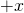direction. Therefore the wall exerts a force on the ball in the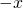direction. The second ball continues with the same momentum component in thedirection, but reverses its-component of momentum, as seen by sketching a diagram of the angles involved and keeping in mind the proportionality between velocity and momentum.

These changes mean the change in momentum for both balls is in thedirection, so the force of the wall on each ball is along thedirection.

Strategy for (b)

Calculate the change in momentum for each ball, which is equal to the impulse imparted to the ball.

Solution for (b)

Let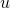be the speed of each ball before and after collision with the wall, and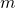the mass of each ball. Choose the-axis and-axis as previously described, and consider the change in momentum of the first ball which strikes perpendicular to the wall.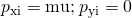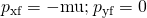Impulse is the change in momentum vector. Therefore the-component of impulse is equal to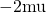and the-component of impulse is equal to zero.

Now consider the change in momentum of the second ball.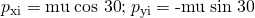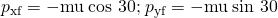It should be noted here that while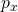changes sign after the collision,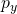does not. Therefore the-component of impulse is equal to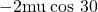and the-component of impulse is equal to zero.

The ratio of the magnitudes of the impulse imparted to the balls is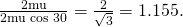Discussion

The direction of impulse and force is the same as in the case of (a); it is normal to the wall and along the negativedirection. Making use of Newton’s third law, the force on the wall due to each ball is normal to the wall along the positive-direction.

Our definition of impulse includes an assumption that the force is constant over the time interval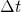. Forces are usually not constant. Forces vary considerably even during the brief time intervals considered. It is, however, possible to find an average effective force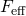that produces the same result as the corresponding time-varying force. (Figure) shows a graph of what an actual force looks like as a function of time for a ball bouncing off the floor. The area under the curve has units of momentum and is equal to the impulse or change in momentum between times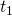and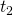. That area is equal to the area inside the rectangle bounded by,, and. Thus the impulses and their effects are the same for both the actual and effective forces.

A graph of force versus time with time along the-axis and force along the-axis for an actual force and an equivalent effective force. The areas under the two curves are equal.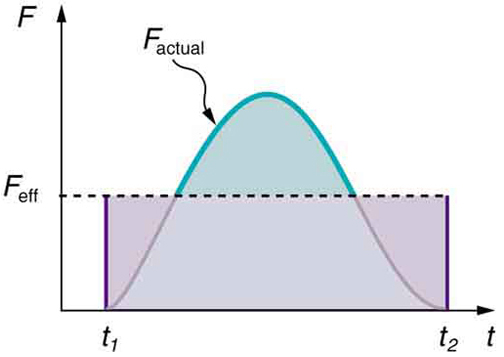Making Connections: Take-Home Investigation—Hand Movement and Impulse

Try catching a ball while “giving” with the ball, pulling your hands toward your body. Then, try catching a ball while keeping your hands still. Hit water in a tub with your full palm. After the water has settled, hit the water again by diving your hand with your fingers first into the water. (Your full palm represents a swimmer doing a belly flop and your diving hand represents a swimmer doing a dive.) Explain what happens in each case and why. Which orientations would you advise people to avoid and why?

Making Connections: Constant Force and Constant Acceleration

The assumption of a constant force in the definition of impulse is analogous to the assumption of a constant acceleration in kinematics. In both cases, nature is adequately described without the use of calculus.

### Section Summary

• Impulse, or change in momentum, equals the average net external force multiplied by the time this force acts: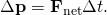• Forces are usually not constant over a period of time.

### Conceptual Questions

Professional Application

Explain in terms of impulse how padding reduces forces in a collision. State this in terms of a real example, such as the advantages of a carpeted vs. tile floor for a day care center.

While jumping on a trampoline, sometimes you land on your back and other times on your feet. In which case can you reach a greater height and why?

Professional Application

Tennis racquets have “sweet spots.” If the ball hits a sweet spot then the player’s arm is not jarred as much as it would be otherwise. Explain why this is the case.

### Problems & Exercises

A bullet is accelerated down the barrel of a gun by hot gases produced in the combustion of gun powder. What is the average force exerted on a 0.0300-kg bullet to accelerate it to a speed of 600 m/s in a time of 2.00 ms (milliseconds)?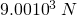Professional Application

A car moving at 10 m/s crashes into a tree and stops in 0.26 s. Calculate the force the seat belt exerts on a passenger in the car to bring him to a halt. The mass of the passenger is 70 kg.

A person slaps her leg with her hand, bringing her hand to rest in 2.50 milliseconds from an initial speed of 4.00 m/s. (a) What is the average force exerted on the leg, taking the effective mass of the hand and forearm to be 1.50 kg? (b) Would the force be any different if the woman clapped her hands together at the same speed and brought them to rest in the same time? Explain why or why not.

a)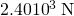toward the leg

b) The force on each hand would have the same magnitude as that found in part (a) (but in opposite directions by Newton’s third law) because the change in momentum and the time interval are the same.

Professional Application

A professional boxer hits his opponent with a 1000-N horizontal blow that lasts for 0.150 s. (a) Calculate the impulse imparted by this blow. (b) What is the opponent’s final velocity, if his mass is 105 kg and he is motionless in midair when struck near his center of mass? (c) Calculate the recoil velocity of the opponent’s 10.0-kg head if hit in this manner, assuming the head does not initially transfer significant momentum to the boxer’s body. (d) Discuss the implications of your answers for parts (b) and (c).

Professional Application

Suppose a child drives a bumper car head on into the side rail, which exerts a force of 4000 N on the car for 0.200 s. (a) What impulse is imparted by this force? (b) Find the final velocity of the bumper car if its initial velocity was 2.80 m/s and the car plus driver have a mass of 200 kg. You may neglect friction between the car and floor.

a)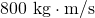away from the wall

b)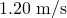away from the wall

Professional Application

One hazard of space travel is debris left by previous missions. There are several thousand objects orbiting Earth that are large enough to be detected by radar, but there are far greater numbers of very small objects, such as flakes of paint. Calculate the force exerted by a 0.100-mg chip of paint that strikes a spacecraft window at a relative speed of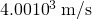, given the collision lasts.

Professional Application

A 75.0-kg person is riding in a car moving at 20.0 m/s when the car runs into a bridge abutment. (a) Calculate the average force on the person if he is stopped by a padded dashboard that compresses an average of 1.00 cm. (b) Calculate the average force on the person if he is stopped by an air bag that compresses an average of 15.0 cm.

(a)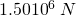away from the dashboard

(b)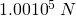away from the dashboard

Professional Application

Military rifles have a mechanism for reducing the recoil forces of the gun on the person firing it. An internal part recoils over a relatively large distance and is stopped by damping mechanisms in the gun. The larger distance reduces the average force needed to stop the internal part. (a) Calculate the recoil velocity of a 1.00-kg plunger that directly interacts with a 0.0200-kg bullet fired at 600 m/s from the gun. (b) If this part is stopped over a distance of 20.0 cm, what average force is exerted upon it by the gun? (c) Compare this to the force exerted on the gun if the bullet is accelerated to its velocity in 10.0 ms (milliseconds).

A cruise ship with a mass of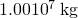strikes a pier at a speed of 0.750 m/s. It comes to rest 6.00 m later, damaging the ship, the pier, and the tugboat captain’s finances. Calculate the average force exerted on the pier using the concept of impulse. (Hint: First calculate the time it took to bring the ship to rest.)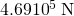in the boat’s original direction of motion

Calculate the final speed of a 110-kg rugby player who is initially running at 8.00 m/s but collides head-on with a padded goalpost and experiences a backward force of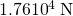for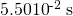.

Water from a fire hose is directed horizontally against a wall at a rate of 50.0 kg/s and a speed of 42.0 m/s. Calculate the magnitude of the force exerted on the wall, assuming the water’s horizontal momentum is reduced to zero.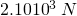away from the wall

A 0.450-kg hammer is moving horizontally at 7.00 m/s when it strikes a nail and comes to rest after driving the nail 1.00 cm into a board. (a) Calculate the duration of the impact. (b) What was the average force exerted on the nail?

Starting with the definitions of momentum and kinetic energy, derive an equation for the kinetic energy of a particle expressed as a function of its momentum.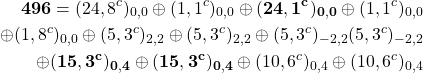A ball with an initial velocity of 10 m/s moves at an angle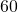above the-direction. The ball hits a vertical wall and bounces off so that it is movingabove the-direction with the same speed. What is the impulse delivered by the wall?

When serving a tennis ball, a player hits the ball when its velocity is zero (at the highest point of a vertical toss). The racquet exerts a force of 540 N on the ball for 5.00 ms, giving it a final velocity of 45.0 m/s. Using these data, find the mass of the ball.

60.0 g

A punter drops a ball from rest vertically 1 meter down onto his foot. The ball leaves the foot with a speed of 18 m/s at an angle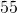above the horizontal. What is the impulse delivered by the foot (magnitude and direction)?

### Glossary

change in momentum
the difference between the final and initial momentum; the mass times the change in velocity
impulse
the average net external force times the time it acts; equal to the change in momentum